Introduction: (written 9-3-06)
This experiment was a stepping-stone on my path to designing an engine-controlled neon light power supply circuit.
The output potential of a typical voltage regulator depends on the voltage of it's ground reference.  This ground reference sinks a mere ~5mA, and can therefore be supplied by the output of a standard op-amp.  Thus, a high-power supply voltage can be controlled by a low-power reference voltage; a function very useful in a variety of applications.
This capability/technique is so deep in my knowledge of EE solutions that it's hard to believe I ever questioned it - it's so obvious.
So if you design circuits and you've never considered this technique - check it out!

Controlling a Voltage Regulator using an Op-Amp

Devin R Ott
Design - completed in August 2004

A voltage regulator performs the function of stabilizing its output voltage to a certain potential difference relative to its ground pin.  The regulator’s supply voltage provides it with output power, while the voltage at the ground pin (VGND) acts as a reference for the device to regulate its output voltage above.  Under normal circumstances, VGND would be tied to the ground potential of the circuit, so if it was a 5 volt regulator, the output voltage would be 5 volts above ground.  However, since the reference at VGND is required to sink only a few milliamps from the ground pin, this potential can theoretically be supplied by the output of an operational amplifier.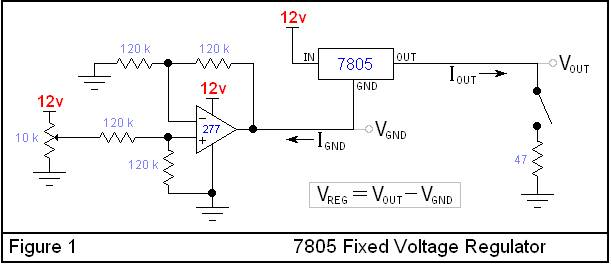The circuit in figure 1 can test this concept using a 7805 positive 5 volt regulator.  The potential at VGND is supplied by the output of a difference amplifier circuit built around the OPA277.   It functions like a non-inverting unity-gain DC amplifier that regulates its output (VGND) according to the voltage at the wiper of the 10k potentiometer.  As VGND increases, VOUT should increase at an equal rate.

The table below compares the data of the unloaded circuit to the data obtained when VOUT was loaded with a 47Ω resistor.  As VGND was adjusted from about 2 volts to 5 volts, the regulated potential difference (VREG) stayed reasonably consistent at about 4.93 volts, and was not affected by loading.  However, the data suggests that when the regulator was loaded with 47Ω, the op-amp’s output voltage (VGND) was slightly weakened by an average of 52mV, causing VOUT to decrease along with it.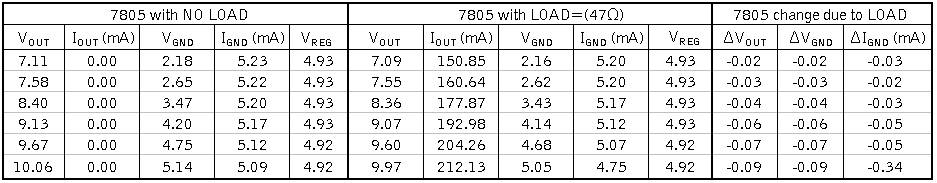The drop in VGND was also accompanied by a slight decrease in IGND by an average of about 40nA, when the regulator was loaded.  The 7805 regulator has a minimum 2 volt drop from the input to output.  As expected, when VOUT got to within 2 volts of the supply voltage, the loading of the regulator began to have an increasing affect on IGND, causing it to decrease dramatically.  Overall, the 5mA of current flowing out of the regulator’s ground pin had little effect on the op-amp’s output voltage (VGND).  For most applications, the slight 50mV fluctuations in VOUT due to loading would be insignificant.

The circuit in Figure 1 showed that the op-amp’s output voltage remained relatively stable when used as the ground reference of a fixed voltage regulator.  Figure 2 illustrates a similar design, using the LM350 adjustable voltage regulator in place of the 7805 fixed regulator.  The VREG of the LM350 is determined by the two resistors R1 and R2, and the current flowing out of the devices adjustment pin (IADG).  This circuit is supplied with 20 volts to provide a larger range of adjustment.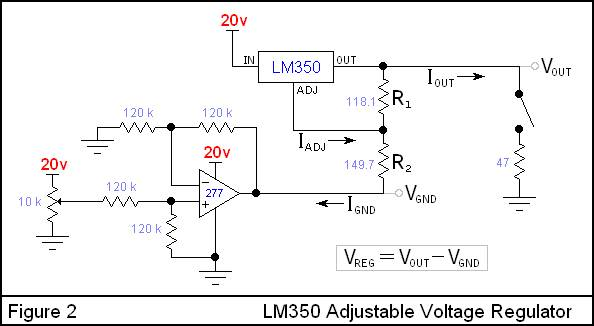The regulated potential difference (VREG) of the LM350 regulator in Figure 1 has been programmed to 2.80 volts.  As seen in the data from the unloaded LM350, the current flowing into the op-amp’s output is 10mA, twice the current of the 7805 circuit.  Despite the increased current flowing into the op-amp’s output, the voltage VGND was not weakened by the loading of the regulator.  In fact, VGND actually increased in response to the regulators load, by about 55mV on average, causing VOUT to do the same.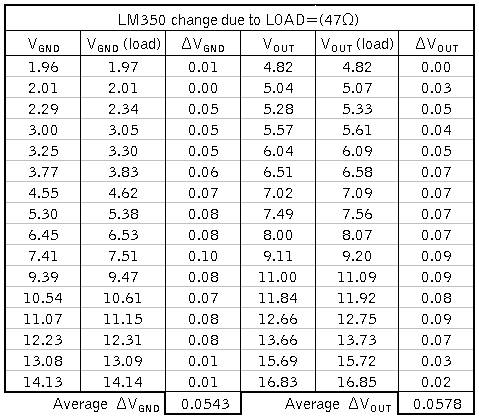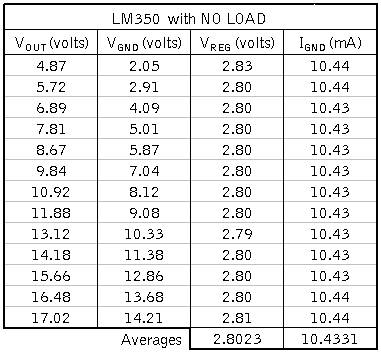As previously stated, the regulated potential difference VREG is determined not only by the two resistors R1 and R2, but also by the current flowing out of the adjustment pin (IADG).  Ohm’s law can be used to derive an equation for IADG in terms of VREG, IGND, R1 and R2.  However, ohm’s law cannot predict how the VIN-VOUT voltage difference will affect the adjustment current.  With R1=120Ω as recommended by the datasheet, the change in IADJ due to the VIN-VOUT potential is negligible, so the output voltage is not affected by the regulators input supply voltage.

For other resistor combinations, such as R1=2kΩ and R2=820Ω, IADJ is dramatically affected by the regulators VIN-VOUT potential difference.  In this case, as VGND changes, so does the VIN-VOUT potential, causing IADJ and thus VREG to change as well.  At the lower end of the range, VREG was 3 volts, and by the upper end of the range, VREG had dropped to 2 volts.

In conclusion, a voltage regulator can be safely adjusted via the ground reference VGND, while maintaining reasonably high VOUT stability relative to the current load (IOUT).

Back to my EE Page

Back to